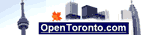# BMI Calculator

 Select metric or standard units: Metric        Standard Your height in centimetres (cm) feet, inches: feet        inches Your body weight in kilograms (kg)pounds (lb): Select rounding: 1 digit after decimal point 2 digits after decimal point 3 digits after decimal point 4 digits after decimal point 5 digits after decimal point

 Other Calculators: Calculate daily calorie intake

## BMI Categories

 Class 3 Obesity more than 40 Class 2 Obesity from 35 - 40 Class 1 Obesity from 30.1 - 34.9 Overweight from 25 - 30 Normal body from 18.5 - 24.9 Underweight body from 16.5 - 18.4 Severely underweight less than 16.5

BMI is a body weight divided by a square of person's height and measured in kg/m2. This calculator provides calculation of your BMI in both metric and USA standard units.

Calculators:
MPG calculator
L/100km fuel calculator
L/100km to MPG calculator
MPG to L/100km conversion
Miles to km conversion
mph to kmh conversion
Gallon to Litre Conversion
Pounds to kilograms conversion
Celsius to Fahrenheit
Fahrenheit to Celsius conversion
Recommended Daily Calorie Calculator
BMI Calculator
Ounces to grams conversion
Yards to mm conversion
Yards to meters Conversion
Feet to meters Conversion
Inches to mm Conversion
Time in Hamilton, Ontario